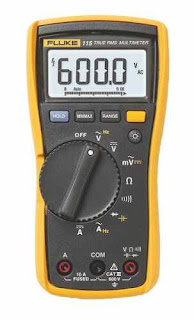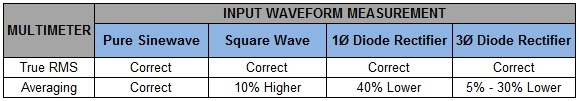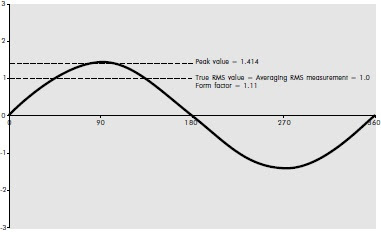## Saturday, August 6, 2011

True RMS Multimeter, as the name suggest, measures the real root mean square value of the input current and voltage. It utilizes an integrated circuit that derives the true RMS of dynamic and complex waveforms of all shapes and sizes. This capability allows the true RMS multimeter to provide accurate measurements regardless of current and voltage waveform (i.e. pure sinewave, square, harmonic-distorted or non-sinusoidal).True RMS Multimeter
The importance of getting an accurate measurement is generally taken for granted. However, in many commercial and industrial installations, the connected loads are usually nonlinear such as solid state controls, variable speed drives and HID lighting. These devices generate harmonics that could severely distort the current and voltage waveform.

Therefore, using the traditional averaging multimeter could lead to inaccurate readings that have costly consequences such as nuisance tripping of circuit breakers due to under measurement. This is because ordinary meters simply measure the average value and multiply it by the so-called form factor to give a calibrated RMS value. Nevertheless, the calibrated RMS will only be equal to the True RMS if the input waveform measured is a perfect sinewave. Otherwise, the differences shown in the table below can be expected.True RMS versus Averaging Multimeter Measurements
The True RMS multimeter has been in the market for at least the past three decades. Unfortunately, due to its relatively expensive price it has yet to surpass the averaging multimeter in terms of orders. Nonetheless, the increase use of electronic devices will eventually lead to making the true RMS multimeter as the first choice for voltage and current measurements.

Root Mean Square (RMS)

The RMS value is basically derived by getting the square of the instantaneous values of the input voltage or current, then, averaging over time, and finally taking the square root. Thus, the term root mean square. The use of the RMS value is to make power calculations simpler by providing the equivalent DC voltage that would produce the same amount of heat in a fixed resistive load.

For a pure sinewave, the peak value is √2 or 1.414 times the RMS value. Meanwhile, the RMS is about 1.111 times the average of the absolute values of the waveform’s magnitude over one cycle. This would also mean that the average is only 0.636 of the peak value. Mathematically, the relationship of the RMS, average and peak values would be like this:

Peak/RMS = √2 or 1.414 (Peak Factor)
RMS/Average = 1.111 (Form Factor)
Average/Peak = 0.636A Perfect Sinewave
The image above shows that when measuring a pure sinewave both multimeter types will give the same RMS readings.  In this case, the ordinary multimeter will simply measure the average value and multiply that value by 1.111 - the form factor. This is the same method that analog meters use.

Applications

A True RMS multimeter is best to use when taking voltage and current measurements in the following:

·         Variable Speed Drives
·         Solid State Controls
·         Power Supplies
·         IT-intensive Environment
·         Industrial Motors
·         Motor and Fan Controls
·         Machine Tool Drives
·         Phase Control Dimmers
·         High Efficiency HVAC Systems

Under Measurement

Under measurement occurs very frequently in modern installations. Average values are usually 40% less than the True RMS. As a result, equipment, wires, cables and their corresponding protective devices such as circuit breakers could be under rated. This mistake could lead to nuisance tripping, malfunctioning motors, or worst case, potential fire hazards. Furthermore, True RMS multimeters are around 20%-30% more than its counterpart – a small price for an accurate and safe voltage and current measurement.

Note: The author has no intention of promoting the multimeter brands shown in this post, and has only embedded the images and video as he finds them helpful to the readers of this site.

References:
Tanuwijaya, F. And Muhammad, F. (2009). True RMS vs. AC Average Rectified Multimeter Readings when a Phase Cutting Speed Control is Used.
West, K. (2001). True RMS – The Only True Measurement. Copper Development Authority